• 哈希函数--2021-07-28
2021-07-28 20:06:53

【题目描述】

设x,y的hash函数为：h=xy+x+y。对于给出的一个h值，问有多少对有序数对（x,y）满足max(x,y)<=h，h,x,y都为非负整数。

【输入格式】

多组测试数据，第一行行为测试点的个数T,接下来每一行一个整数h.

【输出格式】

一共T行，每行一个整数，分别表示有多少组（x,y）满足要其对应的h值。

【输入样例】

3

1

3

4

【输出样例】

3

2

【数据规模与约定】

对于30%的数据，0<=h<=2*10^4,T<=1000.

对于100%的数据，0<=h<=10^8,T<=10000.

【算法分析】

对于h=xy+x+y这个式子可以变换h+1=(x+1)(y+1).

令s=h+1,a=x+1,b=y+1;

于是题目就变成了：对于式子s=ab有多少组（a,b）满足要求。

这个时候只要对s进行质因数分解，然后统计每个质因子个数，答案就出来了：

ans=ans*(filli+1);(filli为分解后第i个质因子的个数）

时间复杂度O（nlogn），如果用优化过的埃氏筛时间复杂度为O（n）

【核心代码

#include<iostream>
#incldue<cstdio>
using namespace std;
int main()
{
for(int i=2;i<=1000;++i)
if(!b[i])
{
s[++tot]=i;
for(int j=2;i*j<=10000;++j)b[i*k]=1;
}
int T;
cin>>T;
while(T--)
{
int ans=1;
int n;
cin>>n;
n++;
for(int i=1;i<=tot;++i)
{
if(n==1)break;
int v=s[i],tot=0;
while(n%v==0)n/v,tot++;
ans*=tot+1;
}
if(n!=1)ans*=2;
printf("%d\n",ans);

}算法 数据结构 推荐算法
更多相关内容
• 哈希函数 一般都直接取模，取模的数一般来说取质数，并且这个数要离2的整次幂尽可能远。可以证明，这样做引起冲突的概率最小。 冲突 拉链法：开一个一维数组，存储所有哈希值，在每一个槽上加一个链，用来存储这个槽...

## 存储结构

1.开放寻址法（y总推荐）
开到题目范围的2-3倍，开放寻址法原理类似于上厕所
2.拉链法
类似于邻接表

## 字符串哈希方式

本质：用一个p进制的数来表示字符串
p进制
1.把字符串看成p进制的数
2,.把p进制的数转化为10进制的数
3.对整个数modQ
这样，就可以把任何一个字符串映射到0~Q-1之间的数了
两个原则
1.不能映射成0
2.人品足够好，不存在冲突
当p=131或13331
Q取成2e64
此时，绝大多数情况下是没有冲突的。
除了循环节，大多数问题上，kmp都打不过字符串哈希

## 哈希表常用操作

算法里常考：添加，查找
如果非要实现删除，也不是真正删掉这个点，而是开一个布尔变量标记

## 哈希函数

一般都直接取模，取模的数一般来说取质数，并且这个数要离2的整次幂尽可能远。可以证明，这样做引起冲突的概率最小。

## 冲突

拉链法：开一个一维数组，存储所有哈希值，在每一个槽上加一个链，用来存储这个槽上有的所有冲突的数。

AcWing 840. 模拟散列表
维护一个集合，支持如下几种操作：
“I x”，插入一个数x；
“Q x”，询问数x是否在集合中出现过；
现在要进行N次操作，对于每个询问操作输出对应的结果。

输入格式
第一行包含整数N，表示操作数量。

接下来N行，每行包含一个操作指令，操作指令为”I x”，”Q x”中的一种。

输出格式
对于每个询问指令“Q x”，输出一个询问结果，如果x在集合中出现过，则输出“Yes”，否则输出“No”。

每个结果占一行。

数据范围
1≤N≤105
−109≤x≤109
输入样例：
5
I 1
I 2
I 3
Q 2
Q 5
输出样例：
Yes
No

拉链法

#include <bits/stdc++.h>
using namespace std;
const int N = 100003;
int h[N], ne[N], e[N], idx;
void myinsert(int x)
{
int k = (x % N + N) % N;
e[idx] = x;
ne[idx] = h[k];
h[k] = idx++;
}
bool myfind(int x)
{
int k = (x % N + N) % N;
for(int i = h[k]; i != -1; i = ne[i])
{
if(e[i] == x)
return true;
}
return false;
}
int main()
{
ios::sync_with_stdio(false);
cin.tie(0);
int n, x;
string s;
memset(h, -1, sizeof h);
cin >> n;
while(n--)
{
cin >> s >> x;
if(s == "I")
myinsert(x);
if(s == "Q")
{
if(myfind(x))
cout << "Yes" << '\n';
else
cout << "No" << '\n';
}
}
return 0;
}



开放寻址法

#include <bits/stdc++.h>
using namespace std;
const int N = 200003;
const int null = 0x3f3f3f3f;
int h[N];
int myfind(int x)
{
int t = (x % N + N) % N;
while(h[t] != null && h[t] != x)
{
t++;
if(t == N)
t = 0;
}
return t;
}
int main()
{
ios::sync_with_stdio(false);
cin.tie(0);
int n, x;
string s;
memset(h, 0x3f, sizeof h);
cin >> n;
while(n--)
{
cin >> s >> x;
if(s == "I")
{
h[myfind(x)] = x;
}
if(s == "Q")
{
if(h[myfind(x)] == null)
cout << "No" << '\n';
else
cout << "Yes" << '\n';
}
}
return 0;
}



注意点：memset是按字节赋值，所以保险的取值是0或者-1

ACWING841 字符串哈希
题目描述
给定一个长度为n的字符串，再给定m个询问，每个询问包含四个整数l1,r1,l2,r2l1,r1,l2,r2，请你判断[l1,r1l1,r1]和[l2,r2l2,r2]这两个区间所包含的字符串子串是否完全相同。

字符串中只包含大小写英文字母和数字。

输入格式
第一行包含整数n和m，表示字符串长度和询问次数。

第二行包含一个长度为n的字符串，字符串中只包含大小写英文字母和数字。

接下来m行，每行包含四个整数l1,r1,l2,r2l1,r1,l2,r2，表示一次询问所涉及的两个区间。

注意，字符串的位置从1开始编号。

输出格式
对于每个询问输出一个结果，如果两个字符串子串完全相同则输出“Yes”，否则输出“No”。

每个结果占一行。

数据范围
1≤n,m≤1051≤n,m≤105
输入样例:
8 3
aabbaabb
1 3 5 7
1 3 6 8
1 2 1 2
输出样例:
Yes
No
Yes

AC代码

#include <bits/stdc++.h>
using namespace std;
const int N = 100010, P = 131;
typedef unsigned long long ULL;
int h[N], p[N];
char x[N];
ULL query(int l, int r)
{
return h[r] - h[l - 1] * p[r - l + 1];
}
int main()
{
ios::sync_with_stdio(false);
cin.tie(0);
int n, m, l1, r1, l2, r2;
h = 0, p = 1;
cin >> n >> m;
cin >> x + 1;
for(int i = 1; i <= n; i++)
{
h[i] = h[i - 1] * P + x[i];
p[i] = p[i - 1] * P;
}
while(m--)
{
cin >> l1 >> r1 >> l2 >> r2;
if(query(l1, r1) == query(l2, r2))
cout << "Yes" << '\n';
else
cout << "No" << '\n';
}
return 0;
}


注意点：p[i]表示p的i次方的值，h[i]表示从1到i的字符串的哈希值，也就是字符串的前缀值。
用unsigned long long来存h和p数组，可以省去modQ这个过程。
公式
对形如 X1X2X3⋯Xn−1XnX1X2X3⋯Xn−1Xn 的字符串,采用字符的ascii 码乘上 P 的次方来计算哈希值。
映射公式 (X1×Pn−1+X2×Pn−2+⋯+Xn−1×P1+Xn×P0)modQ

对于字符串x1x2x3
h = x1
h = h * P + x2 = x1 * p + x2
h = h * p + x3 = x1 * p^2 + x2 * p + x3
现在我们想求x2x3的哈希值，可以记成是x2 * p+x3。可见这个表达式和h只差一个x1 * p^2。我们可以把h乘以p的二次方，而2 = 3 - 2 + 1（r - l + 1），1 = 2 - 1（l - 1）。
故从2到3的字符串哈希值为：h - h[2-1] * p[3-2+1]

推到一般情形，从l到r的字符串哈希值为：h[r] - h[l-1] * p[r-l+1]

展开全文散列表 数据结构
• 数据结构与算法-哈希表与字符串经典例题汇总

### 【数据结构与算法-哈希表与字符串经典例题汇总】

• 基础点击：
• 概念：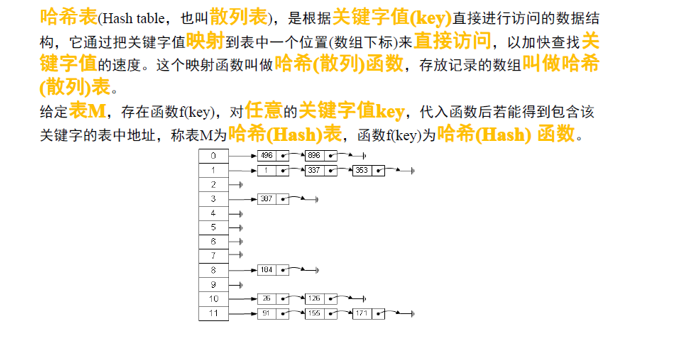• 哈希函数

#include <stdio.h>
#include <string>

int int_func(int key, int table_len){
return key % table_len;
}

int string_func(std::string key, int table_len){
int sum = 0;
for (int i = 0; i < key.length(); i++){
sum += key[i];
}
return sum % table_len;
}

int main(){
const int TABLE_LEN = 10;
int hash_map[TABLE_LEN] = {0};
hash_map[int_func(99999995, TABLE_LEN)]++;
hash_map[int_func(5, TABLE_LEN)]++;
hash_map[string_func("abc", TABLE_LEN)]++;
hash_map[string_func("bac", TABLE_LEN)]++;
for (int i = 0; i < TABLE_LEN; i++){
printf("hash_map[%d] = %d\n", i, hash_map[i]);
}
return 0;
}


• 哈希排序：
#include <stdio.h>

int main(){
int random = {999, 1, 444, 7, 20, 9, 1, 3, 7, 7};
int hash_map = {0};
for (int i = 0; i < 10; i++){
hash_map[random[i]]++;
}
for (int i = 0; i < 1000; i++){
for (int j = 0; j < hash_map[i]; j++){
printf("%d\n", i);
}
}
return 0;
}

• 字符串哈希
#include <stdio.h>
#include <string>

int main(){
int char_map = {0};
std::string str = "abcdefgaaxxy";

for (int i = 0; i < str.length(); i++){
char_map[str[i]]++;
}

for (int i = 0; i < 128; i++){
if (char_map[i] > 0){
printf("[%c][%d] : %d\n", i, i, char_map[i]);
}
}
return 0;
}

• 哈希表插入问题：哈希冲突->拉链法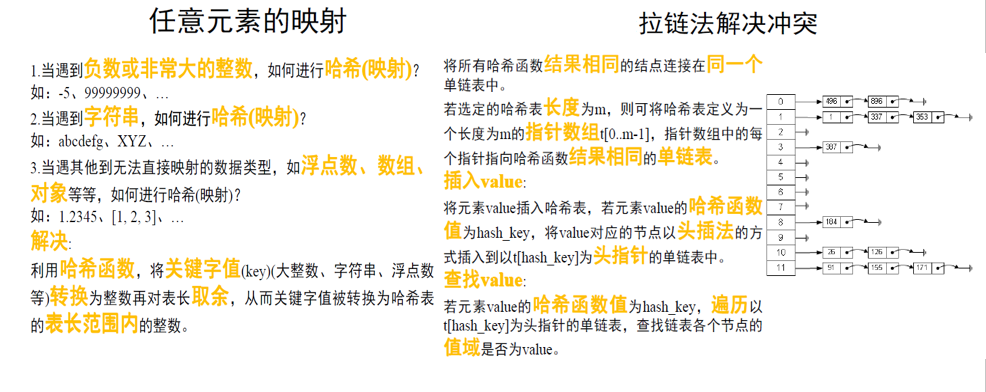• 拉链法


#include <stdio.h>
#include <vector>

struct ListNode {
int val;
ListNode *next;
ListNode(int x) : val(x), next(NULL) {}
};

int hash_func(int key, int table_len){
return key % table_len;
}

void insert(ListNode *hash_table[], ListNode *node, int table_len){
int hash_key = hash_func(node->val, table_len);
node->next = hash_table[hash_key];
hash_table[hash_key] = node;
}

bool search(ListNode *hash_table[], int value, int table_len){
int hash_key = hash_func(value, table_len);
return true;
}
}
return false;
}

int main(){
const int TABLE_LEN = 11;
ListNode *hash_table[TABLE_LEN] = {0};
std::vector<ListNode *> hash_node_vec;
int test = {1, 1, 4, 9, 20, 30, 150, 500};
for (int i = 0; i < 8; i++){
hash_node_vec.push_back(new ListNode(test[i]));
}
for (int i = 0; i < hash_node_vec.size(); i++){
insert(hash_table, hash_node_vec[i], TABLE_LEN);
}
printf("Hash table:\n");
for (int i = 0; i < TABLE_LEN; i++){
printf("[%d]:", i);
}
printf("\n");
}
printf("\n");
printf("Test search:\n");
for (int i = 0; i < 10; i++){
if (search(hash_table, i, TABLE_LEN)){
printf("%d is in the hash table.\n");
}
else{
printf("%d is not in the hash table.\n");
}
}
return 0;
}

• 哈希map 与 STL map

## 典例1、最长回文串（easy）

• 题目描述：409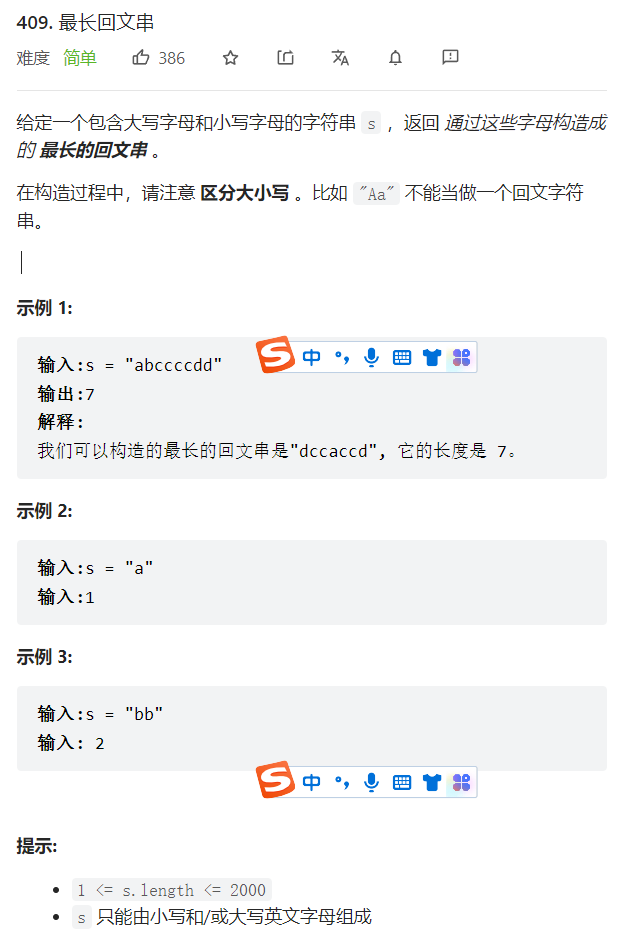• 思路：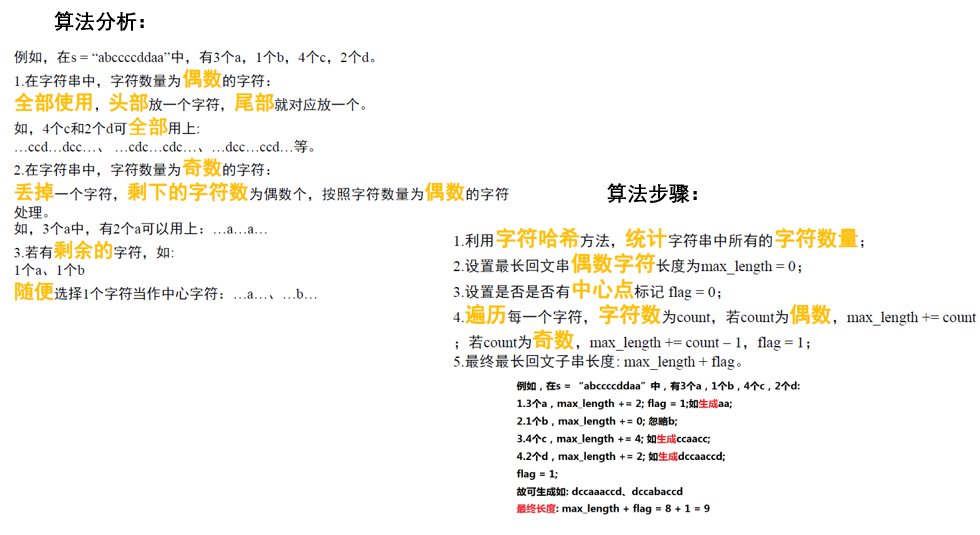• LeetCode提交OJ测试链接:

• OJ测试代码实现：

class Solution {
public:
int longestPalindrome(string s) {
int char_map={0};  // 声明128位字符哈希表
int max_length = 0 ;// 回文字符串偶数部分的最大长度
int flag = 0;   // 是否有中心点
for(int i=0; i< s.length(); i++){
char_map[s[i]]++; // 遍历字符，重复出现的计数
}
for(int i=0;i<128;i++){
if(char_map[i]%2==0){ // 字符出现为偶数
max_length += char_map[i];
}
else{ // 字符出现为奇数
max_length += char_map[i]-1;
flag = 1; // 回文串有中心点
}
}

return flag + max_length;  // 返回值 最大长度+中心点
}
};


python3

class Solution:
def longestPalindrome(self, s: str) -> int:
char_map = {}  #字典来存储每个字母出现的个数
max_length = 0 # 偶数位字符串的最大回文长度
flag = 0  # 是否有中心点（奇数）
for word in s:  # 遍历统计每个字母出现的个数
if word in char_map:
char_map[word] +=1
else:
char_map[word] = 1
for key,value in char_map.items():# 遍历统计每个字母出现的个数奇偶，判断是否有中心点，以及回文的长度
if value % 2 == 0: # 出现偶数次
max_length += value
else:
max_length += value-1  # 出现奇数次
flag = 1

return max_length + flag

• 可本地运行测试的完整代码：




## 典例2、词语模式（easy）

• 题目描述：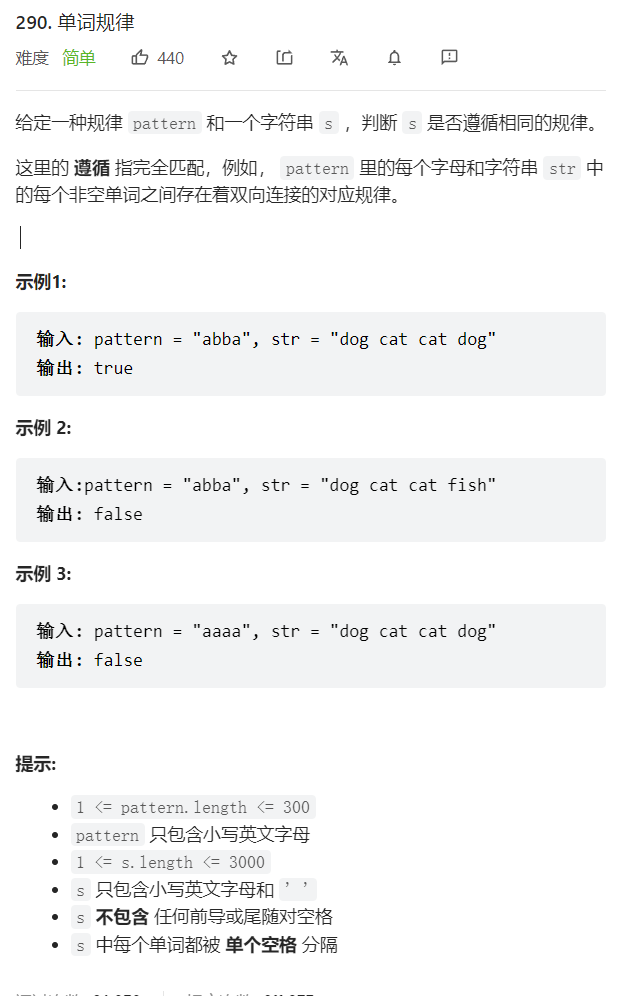• 思路：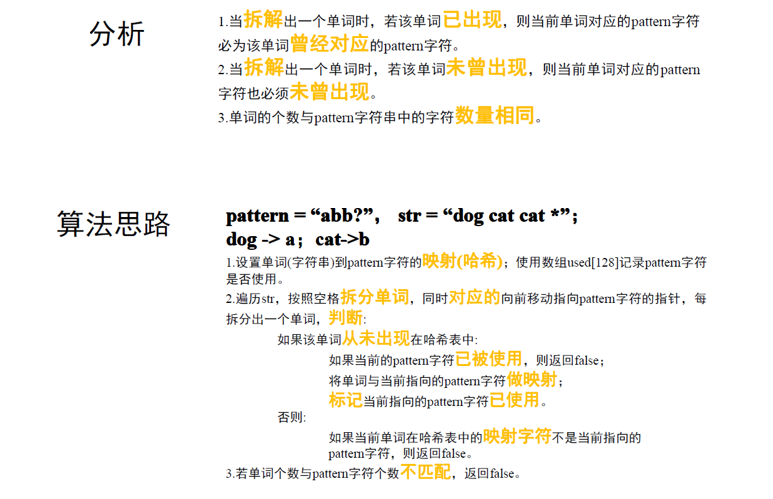• LeetCode提交OJ测试链接:

• OJ测试代码实现：

cpp

class Solution {
public:
bool wordPattern(std::string pattern, std::string str) {
std::map<std::string, char> word_map; // 创建单词到pattern 的映射
char used = {0}; // 已经被映射的字符
std::string word;  //  临时保存拆分出来的单词
int pos = 0;  // 指向当前的pattern字符
str.push_back(' ');  //  使用 " " 拆分新的单词
for (int i = 0; i < str.length(); i++){
if (str[i] == ' '){  //  遇到 " " 拆分新的单词
if (pos == pattern.length()){  // 若拆分新的单词，但是pattern已无字符
return false;
}
if (word_map.find(word) == word_map.end()){
if (used[pattern[pos]]){  // 当前的pattern字符已经使用过
return false;
}
word_map[word] = pattern[pos];
used[pattern[pos]] = 1;
}
else{
if (word_map[word] != pattern[pos]){  //若简历的pattern字符 与单词位置频数不匹配
return false;
}
}
word = "";  //完成一个单词的插入和查询后，清空word
pos++; // 指向pattern字符的指针向前移动
}
else{
word += str[i];
}
}
if (pos != pattern.length()){  // 若是有多余的pattern字符
return false;
}
return true;
}
};


python

class Solution:
def wordPattern(self, pattern: str, s: str) -> bool:
char2word = {}  # dict()
word2char={}
words = s.split() # 将单词进行分隔
if len(words) != len(pattern):# 排除个数不匹配的存在
return False
for char,word in zip(pattern,words): # zip()将对象中对应的元素打包成一个个元组，然后返回由这些元组组成的列表。
if word in word2char and word2char[word] != char:
return False
if char in char2word and char2word[char] != word:
return False
word2char[word] = char
char2word[char] = word
return True

• 可本地运行测试的完整代码：



## 典例3、字母异位词分组（medium）

• 题目描述：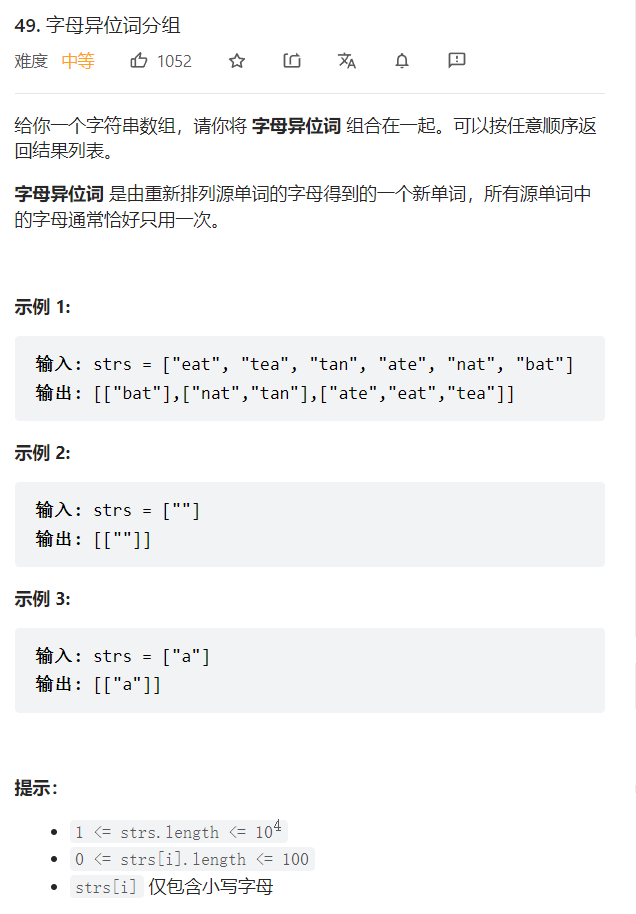• 思路：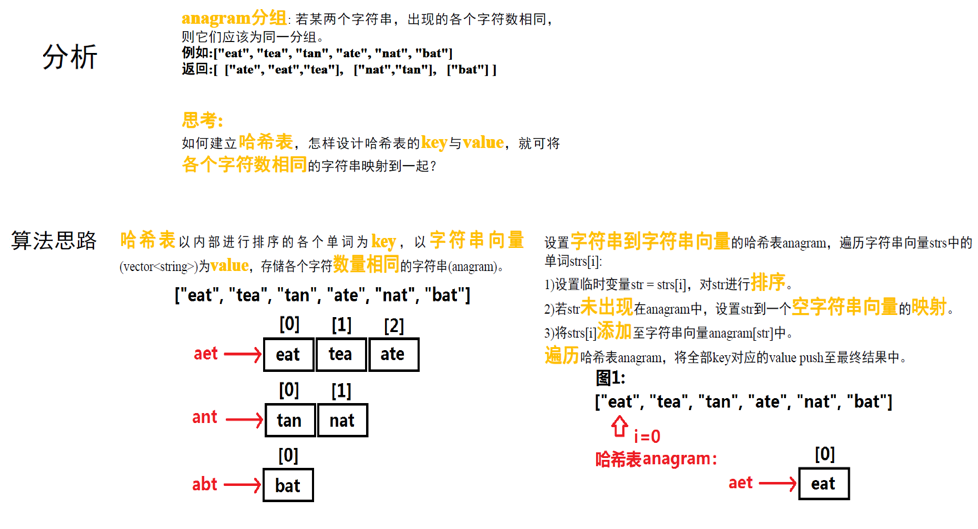• LeetCode提交OJ测试链接:

• OJ测试代码实现：

cpp

见下完整代码


python

class Solution:
def groupAnagrams(self, strs: List[str]) -> List[List[str]]:
mp = collections.defaultdict(list) # 声明mp变量

for st in strs:
key = "".join(sorted(st))
'''由于互为字母异位词的两个字符串包含的字母相同，因此对两个字符串分别进行排序之后得到的字符串一定是相同的，故可以将排序之后的字符串作为哈希表的键'''
mp[key].append(st) # 排序相同的字符串添加到一个 key 下的value中，不同的分开

return list(mp.values()) # 最终返回整个hash—mp 的value值

• 可本地运行测试的完整代码：
#include <stdio.h>

#include <vector>
#include <string>
#include <map>
#include <algorithm>

class Solution {
public:
std::vector<std::vector<std::string> > groupAnagrams(
std::vector<std::string>& strs) {
std::map<std::string, std::vector<std::string> > anagram;
std::vector<std::vector<std::string> > result; // 存储最终的结果
for (int i = 0; i < strs.size(); i++){// 遍历各个单词
std::string str = strs[i];   // 设置临时变量，以进行排序（不改变原来的字母顺序）
std::sort(str.begin(), str.end()); // 内部对临时变量进行排序的字符作为 key ,字符串向量为value
if (anagram.find(str) == anagram.end()){ // 若无法在 anagram 中找到 临时变量
std::vector<std::string> item; // 设置一个空的字符串向量
anagram[str] = item; // 以排序后的strs[i] 作为key
}
anagram[str].push_back(strs[i]);// 在对应的字符串向量中push结果
}
std::map<std::string, std::vector<std::string> > ::iterator it;
for (it = anagram.begin(); it != anagram.end(); it++){ // 遍历哈希表，将哈希表的value push进入最终的结果
result.push_back((*it).second);
}
return result;
}
};

int main(){
std::vector<std::string> strs;
strs.push_back("eat");
strs.push_back("tea");
strs.push_back("tan");
strs.push_back("ate");
strs.push_back("nat");
strs.push_back("bat");
Solution solve;
std::vector<std::vector<std::string> > result
= solve.groupAnagrams(strs);
for (int i = 0; i < result.size(); i++){
for (int j = 0; j < result[i].size(); j++){
printf("[%s]", result[i][j].c_str());
}
printf("\n");
}
return 0;
}



## 典例4、无重复字符的最长子串（medium）

• 题目描述：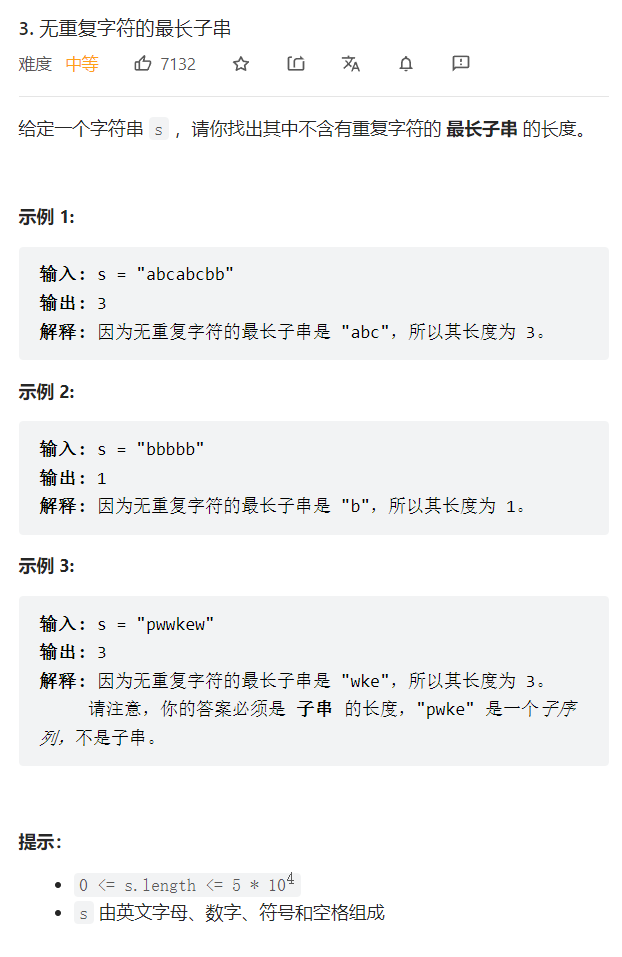• 思路：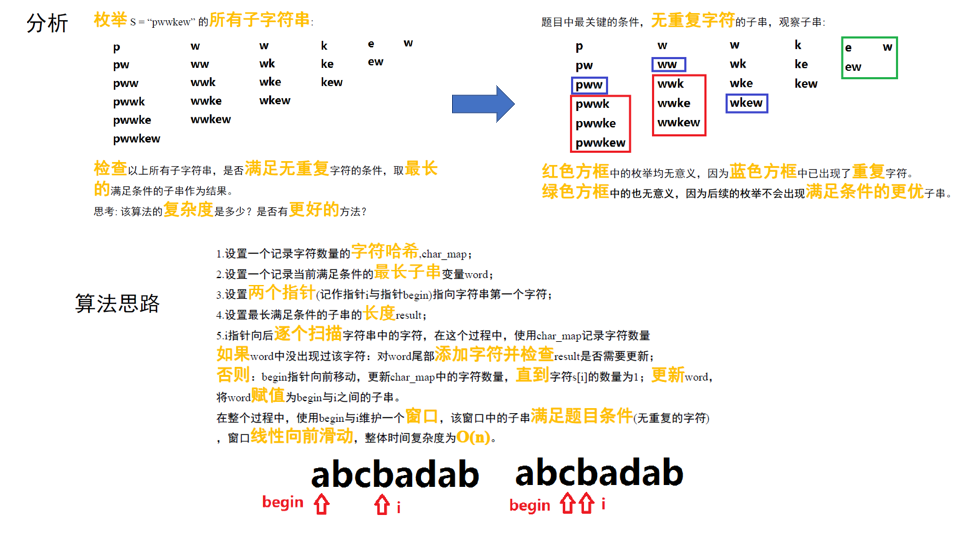• LeetCode提交OJ测试链接:

• OJ测试代码实现：

cpp




python



• 可本地运行测试的完整代码：
#include <stdio.h>

#include <string>
class Solution {
public:
int lengthOfLongestSubstring(std::string s) {
int begin = 0;
int result = 0;
std::string word = "";
int char_map = {0};
for (int i = 0; i < s.length(); i++){
char_map[s[i]]++;
if (char_map[s[i]] == 1){
word += s[i];
if (result < word.length()){
result = word.length();
}
}
else{
while(begin < i && char_map[s[i]] > 1){
char_map[s[begin]]--;
begin++;
}
word = "";
for (int j = begin; j <= i; j++){
word += s[j];
}
}
}
return result;
}
};

int main(){
Solution solve;
printf("%d\n", solve.lengthOfLongestSubstring(s));
return 0;
}



## 典例5、无重复的DNA序列（medium）

• 题目描述：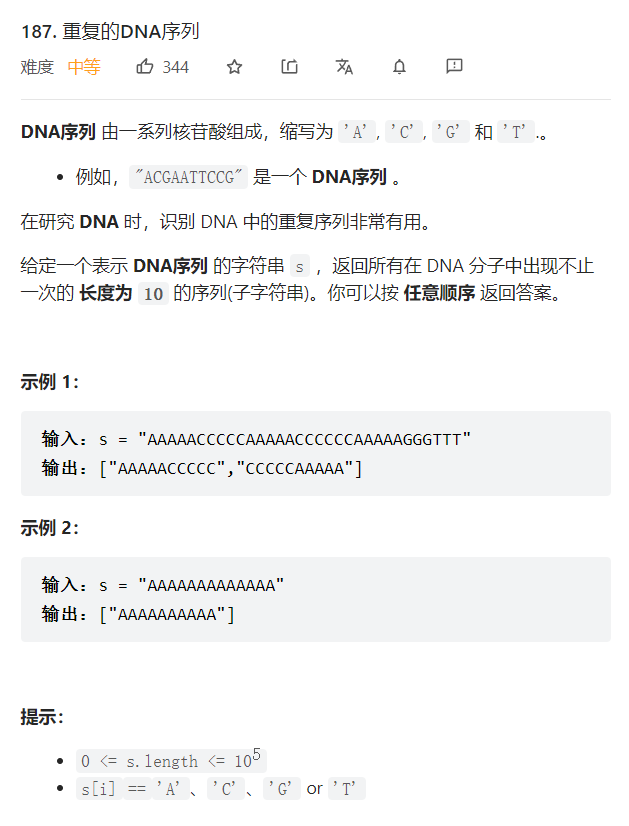• 思路：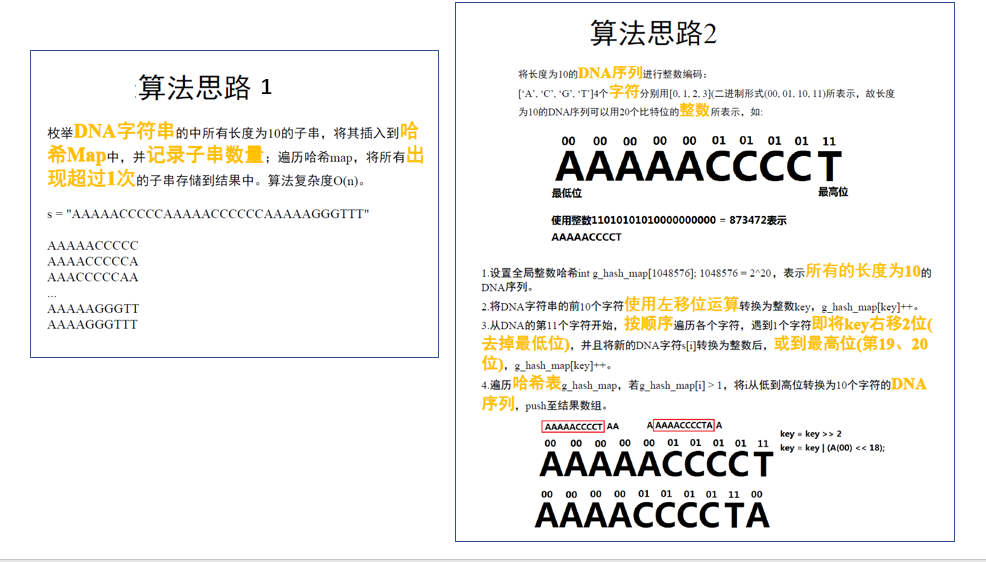• LeetCode提交OJ测试链接:

• OJ测试代码实现：

cpp




python



• 可本地运行测试的完整代码：

方法 1：

#include <stdio.h>

#include <vector>
#include <string>
#include <map>

class Solution {
public:
std::vector<std::string> findRepeatedDnaSequences(std::string s) {
std::map<std::string, int> word_map;
std::vector<std::string> result;
for (int i = 0; i < s.length(); i++){
std::string word = s.substr(i, 10);
if (word_map.find(word) != word_map.end()){
word_map[word] += 1;
}
else{
word_map[word] = 1;
}
}
std::map<std::string, int> ::iterator it;
for (it = word_map.begin(); it != word_map.end(); it++){
if (it->second > 1){
result.push_back(it->first);
}
}
return result;
}
};

int main(){
std::string s = "AAAAACCCCCAAAAACCCCCCAAAAAGGGTTT";
Solution solve;
std::vector<std::string> result = solve.findRepeatedDnaSequences(s);
for (int i = 0; i < result.size(); i++){
printf("%s\n", result[i].c_str());
}
return 0;
}



方法 2：

#include <stdio.h>

#include <vector>
#include <string>

int g_hash_map = {0};

std::string change_int_to_DNA(int DNA){
static const char DNA_CHAR[] = {'A', 'C', 'G', 'T'};
std::string str;
for (int i = 0; i < 10; i++){
str += DNA_CHAR[DNA & 3];
DNA = DNA >> 2;
}
return str;
}

class Solution {
public:
std::vector<std::string> findRepeatedDnaSequences(std::string s) {
std::vector<std::string> result;
if (s.length() < 10){
return result;
}
for (int i = 0; i < 1048576; i++){
g_hash_map[i] = 0;
}
int char_map = {0};
char_map['A'] = 0;
char_map['C'] = 1;
char_map['G'] = 2;
char_map['T'] = 3;
int key = 0;
for (int i = 9; i >= 0; i--){
key = (key << 2) + char_map[s[i]];
}
g_hash_map[key] = 1;
for (int i = 10; i < s.length(); i++){
key = key >> 2;
key = key | (char_map[s[i]] << 18);
g_hash_map[key]++;
}
for (int i = 0; i < 1048576; i++){
if (g_hash_map[i] > 1){
result.push_back(change_int_to_DNA(i));
}
}
return result;
}
};

int main(){
std::string s = "AAAAACCCCCAAAAACCCCCCAAAAAGGGTTT";
Solution solve;
std::vector<std::string> result = solve.findRepeatedDnaSequences(s);
for (int i = 0; i < result.size(); i++){
printf("%s\n", result[i].c_str());
}
return 0;
}



## 典例6、最小窗口子串（hard）

• 题目描述：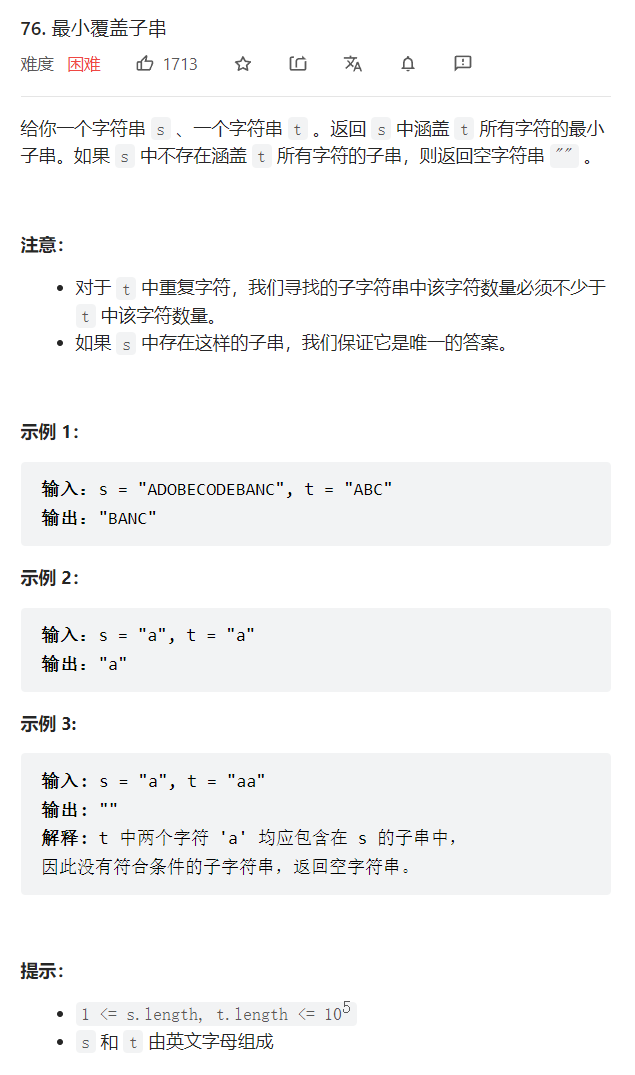• 思路：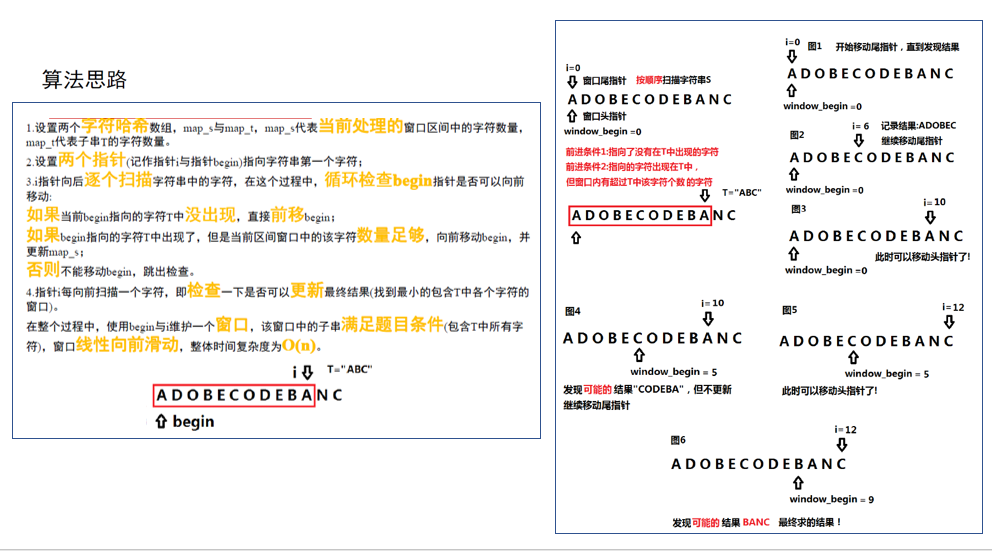• LeetCode提交OJ测试链接:

• OJ测试代码实现：

cpp




python



• 可本地运行测试的完整代码：
#include <stdio.h>

#include <string>
#include <vector>

class Solution {
private:
bool is_window_ok(int map_s[], int map_t[], std::vector<int> &vec_t){
for (int i = 0; i < vec_t.size(); i++){  //利用vec_t遍历t中出现的字符
if (map_s[vec_t[i]] < map_t[vec_t[i]]){ // 如果s出现该字符的数量小于t中出现该字符的数量
return false;
}
}
return true;
}
public:
std::string minWindow(std::string s, std::string t) {
const int MAX_ARRAY_LEN = 128; // char 0-127，利用数组下标记录字符个数
int map_t[MAX_ARRAY_LEN] = {0}; //记录字符串各字符个数
int map_s[MAX_ARRAY_LEN] = {0}; // 记录s字符串各字符个数
std::vector<int> vec_t;  // 记录t字符串中有哪些字符
for (int i = 0; i < t.length(); i++){ // 遍历t，记录s字符串个字符个数
map_t[t[i]]++;
}
for (int i = 0; i < MAX_ARRAY_LEN; i++){ //遍历，将字符串t中出现的字符存储到vec_t中
if (map_t[i] > 0){
vec_t.push_back(i);
}
}

int window_begin = 0; //最小窗口起始指针
std::string result; //最小窗口对应的字符串
for (int i = 0; i < s.length(); i++){  // i代表了窗口的尾指针
map_s[s[i]]++; //将尾指针指向的字符添加到表示窗口的map中
while(window_begin < i){  //窗口的头指针不能超过尾指针
char begin_ch = s[window_begin];
if (map_t[begin_ch] == 0){ //如果当前头指针指向的字符没有在字符串t中出现
window_begin++; // 窗口头指针前移
}
else if	(map_s[begin_ch] > map_t[begin_ch]){ //头指针指向的字符出现在T中，窗口内有超过T中该字符个数的字符
map_s[begin_ch]--; //头指针前移了，它指向的字符减少1个
window_begin++;//窗口头指针前移
}
else{
break; // 除了1.2两个条件，其他情况都跳出循环
}
}
if (is_window_ok(map_s, map_t, vec_t)){  //检查此时的窗口是否包含字符串t
int new_window_len = i - window_begin + 1; //计算新字符串长度
if (result == "" || result.length() > new_window_len){ //结果字符串为空或者当前窗口字符串更小的时候更新结果
result = s.substr(window_begin, new_window_len);  // 替换窗口所对应的字符串
}
}
}
return result;
}
};

int main(){

Solution solve;
printf("%s\n", result.c_str());
printf("%s\n", result.c_str());
result = solve.minWindow("aa", "aa");
printf("%s\n", result.c_str());

return 0;
}


展开全文数据结构
• 昨天我们对哈希的基础知识有了一定的了解，并已经知道了如何求子串、拼接子串的哈希值，今天我们就这两个操作分析一些基础例题，加深理解和掌握。 例题一：子串查找 LOJ #103. 子串查找 显然这是一道kmp算法的模板题...

### 文章目录

哈希前置知识请戳这里-> 哈希绪论

昨天我们对哈希的基础知识有了一定的了解，并已经知道了如何求子串、拼接子串的哈希值，今天我们就这两个操作分析一些基础例题，加深理解和掌握。

## 例题一：子串查找

LOJ #103. 子串查找
显然这是一道kmp算法的模板题

朴素的做法是枚举文本串的每一个位置作为模式串开始比较的位置。设枚举到主串的位置是 i i N N 为主串（即输入的第一行）的长度， M M 为模式串（即输入的第二行）的长度， s a sa 为主串， s b sb 为模式串，则当且仅当 s a sa i i 到第 i + M − 1 i+M-1 与模式串一一匹配，才能使 a n s + 1 ans+1 。这样在最坏情况下（比如 s a 、 s b sa、sb 所有字符均为 a a ），复杂度达到了 O ( N M ) O(NM) ，是不可接受的。现在我们考虑用哈希处理，那么可以将第二步比较的操作复杂度降低到 O ( 1 ) O(1) ，因此只要先预处理出 s a sa 的前缀哈希表，则当我们枚举每一个位置 i i 时，利用前缀表获得区间 [ i , i + M − 1 ] [i,i+M-1] 子串的哈希值，再与 s b sb 的哈希值相比较即可，总体复杂度就降低到了 O ( N + M ) O(N+M) ，已经和kmp算法一样优秀了就是常数有点大
下面来看代码：

#include<stdio.h>
#include<string.h>
#define ll long long
#define N 1000000007
#define M 14371003
#define max 1000005
char s[max], ss[max];
int a[max], p[max];
int main()
{
scanf("%s%s", s + 1, ss + 1);
int len = strlen(ss + 1), ans = 0, cnt = 0, l = strlen(s + 1);
if (len > l) { printf("0"); return 0; }
a = s, p = 1, p = M;
for (int i = 1; i <= len; i++)
ans = ((ll)ans * M + ss[i]) % N;//获得sb的哈希值
for (int i = 2; i <= l; i++)
{
a[i] = ((ll)a[i - 1] * M + s[i]) % N;
p[i] = (ll)p[i - 1] * M % N;
}
for (int i = 1; i <= l + 1 - len; i++)
if (((a[i + len - 1] - (ll)a[i - 1] * p[len]) % N + N) % N == ans)cnt++;
//查询子串哈希值公式
printf("%d", cnt);
return 0;
}


接下来我们看两道道有难度的题目

## 例题二：字符串的删除操作

LOJ #2823. 「BalticOI 2014 Day 1」三个朋友
这道题我们很容易想到利用逆向思维解决：枚举每一个删除的位置，然后检查删除后的字符串是否由两个完全相同的字符串构成，而判断字符串是否相同就可以利用哈希的思想了。

首先，输入的字符串长度一定要是奇数（删掉一个字符是长度是偶数），否则直接输出无解。稍微修改一下昨天拼接字符串的公式就得到了在区间 [ l , r ] [l,r] 删除位置 x x 对应的字符后子串的哈希值公式： a n s = g e t ( l , x − 1 ) ∗ b a s e r − x + g e t ( x + 1 , r ) ans=get(l,x-1)*base^{r-x}+get(x+1,r) 相当于是把 [ l , x − 1 ] 、 [ x + 1 , r ] [l,x-1]、[x+1,r] 两段字符串拼接起来。然后就是需要注意若删除的位置是在原字符串前一半位置，则比较 h a s h ( [ 1 , x − 1 ] + [ x + 1 , l e n 2 ] ) hash([1,x-1]+[x+1,\frac{len}{2}]) h a s h ( [ l e n 2 + 1 , l e n ] ) hash([\frac{len}{2}+1,len]) 是否相等，其中 l e n len 为原字符串的长度， x x 在后半段同理。（其实就是分类讨论 x x 的位置）
最后只要根据符合条件的 x x 的数量来输出多解一解还是无解（需要考虑 x x 不同但字符串相同的情况）
总体复杂度 O ( N ) O(N)

下面是代码：

#include<stdio.h>
#include<string.h>
#include<algorithm>
#define N 1000000007
#define M 14371003
#define ll long long
int ans, p;
int gethash(int l, int r)
{
return ((ans[r] - (ll)ans[l - 1] * p[r - l + 1]) % N + N) % N;
}
int del(int l, int r, int x)//删除操作
{
return ((ll)gethash(l, x - 1) * p[r - x] + gethash(x + 1, r)) % N;
}
int main()
{
int n, f = 0, res, t1, t2, t3, t4; char s;//f标记是否出现过符合条件的x
scanf("%d%s", &n, s);
ans = s, p = 1;
for (int i = 1; i ^ n; i++)
{
ans[i] = ((ll)ans[i - 1] * M + s[i]) % N;
p[i] = (ll)p[i - 1] * M % N;
}
if (n & 1)//只要长度为奇数才可能有解
{
t1 = (n >> 1), t2 = n - 1, t3 = t1 - 1, t4 = t1 + 1;
for (int i = 0; i ^ t4; i++)//枚举前一半
if (!(del(0, t1, i) ^ gethash(t4, t2)))//哈希值相等
{
if (f && (del(0, t2, i) ^ del(0, t2, res)))
{//符合条件的x已经出现且与之前得到的字符串不同则输出多解
printf("NOT UNIQUE");
return 0;
}
f = 1, res = i;
}
for (int i = t4; i ^ n; i++)//枚举后一半
{
if (!(gethash(0, t3) ^ del(t1, t2, i)))
{
if (f && (del(0, t2, i) ^ del(0, t2, res)))
{
printf("NOT UNIQUE");
return 0;
}
f = 1, res = i;
}
}
}
if (!f)//未出现符合条件的位置x或者原字符串长度为偶数则输出无解
{
printf("NOT POSSIBLE");
return 0;
}
if (res <= t1)printf("%s", s + 1 + t1);//x出现在前一半
else//x出现在后一半
{
s[t1] = '\0';
printf("%s", s);
}
return 0;
}


## 例题三：字符串合并操作的应用

牛客 白兔的字符串
对于循环同构，我们可以先预处理出字符串 T T 所有循环同构字符串的哈希值，而每一个循环同构字符串都由区间 [ i + 1 , l e n T ] 、 [ 1 , i ] [i+1,len_T]、[1,i] 拼接而成，可以利用子串拼接的公式进行求解。接着对于每一个给出的 S S 枚举所有起点 i i ，检查子串 [ i , i + l e n T − 1 ] [i,i+len_T-1] 的值是否在哈希表中出现过。当然是先对哈希表进行排序，之后二分查找哈希值即可。总复杂度 O ( n ∗ l e n T ∗ l o g l e n T ) O(n*len_T*loglen_T) 数据比较毒瘤，换了好几个质数
代码：

#include <stdio.h>
#include <string.h>
#include <algorithm>
#define ll long long
#define N 2147483587
#define M 25165843
char s;
int hash, base, rev;
int get(int l, int r)
{
return ((hash[r] - (ll)hash[l - 1] * base[r - l + 1]) % N + N) % N;
}
int merge(int l1, int r1, int l2, int r2)//子串合并操作
{
return ((ll)get(l1, r1) * base[r2 - l2 + 1] + get(l2, r2)) % N;
}
int main()
{
scanf("%s", s + 1);
int len = strlen(s + 1), n; base = 1;
for (int i = 1; i <= len; ++i)
{
hash[i] = ((ll)hash[i - 1] * M + s[i]) % N;
base[i] = ((ll)M * base[i - 1]) % N;
}
for (int i = 1; i < len; ++i)
rev[i] = merge(i + 1, len, 1, i);//合并区间[i+1,len_T]、[1,i]得到同构子串
rev[len] = hash[len];
std::sort(rev + 1, rev + len + 1);//排序，方便二分查找
scanf("%d", &n);
while (n--)
{
int ans = 0;
scanf("%s", s + 1);
int lens = strlen(s + 1);
if (lens < len) { puts("0"); continue; }
for (int i = 1; i <= lens; ++i)
hash[i] = ((ll)hash[i - 1] * M + s[i]) % N;
lens -= len - 1;
for (int i = 1; i <= lens; ++i)//枚举每一个起始位置
if (std::binary_search(rev + 1, rev + len + 1, get(i, i + len - 1)))++ans;
//在哈希表中能找到对应的哈希值，那么答案加一
printf("%d\n", ans);
}
return 0;
}


今天的例题到这里就结束了

明天更新二维哈希、自然溢出以及二分哈希表
不见不散

展开全文字符串 算法 数据结构 hash
• 在数据结构中，哈希函数是用来将一个字符串（或任何其他类型）转化为小于哈希表大小且大于等于零的整数。一个好的哈希函数可以尽可能少地产生冲突。一种广泛使用的哈希函数算法是使用数值33，假设任何字符串都是基于...
• 已知：无符号整数的范围0~2的32次方-1，现在有四十亿个无符号整数，求其中出现次数最多的那个数。...把每个数求出一个哈希值，哈希值再模上100，把得到相同的余数的放到一个文件里面。每个文件大小是32G/100java
• 在某种程度上，散列是与排序相反的一种操作，排序是将集合中的元素按照某种方式比如字典顺序排列在一起，而散列通过计算哈希值，打破元素之间原有的关系，使集合中的元素按照散列函数的分类进行排列。 在介绍一些...java
•      哈希表通过在value的存储位置和它的key之间建立一个确定的对应关系f（这个对应关系称为哈希函数），使每个key与数据结构中的一个唯一的存储位置相对应。      一般哈希表都是用来快速判断一个元素是否...
• Redis五种数据结构之Hash(哈希) 常用函数及案例 Redis五种数据结构分别是： String: Key-Value(set key value/get key) Hash: key-filedValue(Map,即key对应Map) List: 有序，可重复 Set: 无序，不可重复 SortedSet...redis hash
• 几种常见的哈希函数（散列函数）构造方法 直接定址法 取关键字或关键字的某个线性函数值为散列地址。 即 H(key) = key 或 H(key) = a*key + b，其中a和b为常数。 比如 除留余数法 取关键字被某个不大于散...
• 之所以要介绍这一小节，主要是几乎所有的哈希函数的应用都离不开定义和性质，也正是因为哈希函数拥有这些性质才在各种场景发挥着优秀的性能。 定义： ，其中输入域为无穷，值域为有限域。 哈希函数的定数据结构 算法
• 声明：本篇博客根据回顾老师上课...创建哈希表时，把关键字为k的元素直接存入地址为H(k)的单元，以后查找关键字为k的元素时，再利用哈希函数计算该元素的存储位置。再按关键字存取元素。Hash中存储的key值都是唯一的。数据结构
• 给定一组查找关键字(32,15,7,11,4,28,56,61,79)，哈希表长为m=12，请按照除留余数法设计一个哈希函数，设每个记录的查找概率相等。 （1）画出按照线性探测再散列处理冲突得到的哈希表（给出求解过程），并计算等概率...数据结构
• 套用这个模板，我们可以求得字符串中，任意一个子串的哈希。 步骤是先求字符串的前缀（后缀）hash（特别要注意求前缀的方法，一个规模小的前缀是如何推到规模大的前缀的: h[i] = h[i - 1] * base + s[i] - ‘a’ - 1...字符串 数据结构
•   给定两个字符串 s 和 t ，编写一个函数来判断 t 是否是 s 的字母异位词。注意：若 s 和 t 中每个字符出现的次数都相同，则称 s 和 t 互为字母异位词。 示例 1: 输入: s = "anagram", t = "nagaram" 输出: true ...python 数据结构与算法 leetcode
•算法 python 数据结构
• 6 消息认证码与抗碰撞哈希函数 本节学习用于保护信息的完整性和真实性的消息认证码（MAC）和抗碰撞的哈希函数（CRHF）。 目录：MAC、构建安全MAC、CBC-MAC、CRHF、HMAC、信息论上MAC。 完整性与真实性...
• 基本思想：首先在元素的关键字K和元素的位置P之间建立一个对应关系f，使得P=f(K),其中f成为哈希函数。 创建哈希表时，把关键字K的元素直接存入地址为f(K)的单元；查找关键字K的元素时利用哈...数据结构 算法 链表
• 给定一组查找关键字(32,15,7,11,4,28,56,61,79)，哈希表长为m=12，请按照除留余数法设计一个哈希函数，设每个记录的查找概率相等。 （1）画出按照线性探测再散列处理冲突得到的哈希表（给出求解过程），并计算等...数据结构
• ## 哈希表经典题目

千次阅读 2021-06-05 12:36:22
1.哈希表 哈希表（hash table），也可译为散列表。...将元素映射到哈希表上就涉及到了哈希函数。 比如要查询某个学生是否在一所学校中，需要将学生姓名映射为哈希表上的索引，通过查询索引下标快速判断该学生
• 哈希集 是集合的实现之一，它是一种存储 不重复值 的数据结构。 因此，通常，使用哈希集来检查该值是否已经出现过。 让我们来看一个例子： 给定一个整数数组，查找数组是否包含任何重复项。 这是一个典型的问题，...
• 1、哈希函数：传入一个字符串返回一个哈希码 数字0~9，字母a~f,长度为16或者32； 这就是哈希函数，mD5哈希。16^16范围。 哈希函数又叫散列函数， 性质：1.输入域是无限的；2.输出域是有限的；3.当你输入参数是...
• ## 哈希题目

千次阅读 2016-01-06 00:13:51
散列表的存储空间是一个下标从0开始的一维数组，散列函数为： H(key) = (keyx3) MOD 7，处理冲突采用线性探测再散列法，要求装填（载）因子为0.7。(1) 请画出所构造的散列表。(2) 分别计算等概率情况下查找成功和...
• 哈希函数定义为 H(key)=key%TSize，其中 TSize 是哈希表的最大大小。 利用只具有正增量的二次探测法来解决冲突。 注意，哈希表的大小最好是素数，如果用户给出的最大大小不是素数，则必须将表大小重新定义为大于...c语言 算法 c++ 数据结构
• 哈希函数与哈希表 哈希函数：一般就是用户传入一个数据，可以是字符串也可以是别的类型。然后该数据作为参数传入哈希函数中，哈希函数会返回一串长度为16或者32，每一个位置都是16进制，可以存放0-9或者是A-F的 ...
• Hash哈希 1.基本概念   Hash,也叫哈希或散列，就是把任意长度...  根据设定的哈希函数H(key)和处理冲突方法将一组关键字映射到一个有限的地址区间上，并以关键字在地址区间中的象作为记录在表中的存储位置，这种...
• 实现方法，建立两个相反的哈希表，即倒排索引的概念，删除时，用最后一项替换删除项，以保持哈希值的连续性。 位图 位图通过其他的数据类型实现，通过其他数组来拼成，对于仅需要两种状态的数组，可以极大的省空间。...
• 模板 核心思想：将字符串看成P进制数，P的经验值是131或13331，取这两个值的冲突... // h[k]存储字符串前k个字母的哈希值, p[k]存储 P^k mod 2^64 // 初始化 p = 1; for (int i = 1; i <= n; i ++ ) { h[i] =字符串 算法 c++
• 哈希函数为：H(key)=keyMOD13,哈希表长为m=15， 设每个记录的查找概率相等，采用以上两种方法处理冲突，查找失败时的平均查找长度各是多少 这题可真得是出到点子上去啦，看视频，找资料，网上有得解释真的是有点...经验分享
• （3）再散列函数法 ，取另外一个函数 （4）链地址法，将冲突以链表形式存储起来，散列表只存储头指针 （5）公共溢出区法，将冲突的放到溢出表中，查找时可以在里面进行顺序查找。 hash函数的构造方法： ......